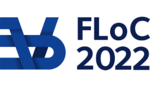FLOC 2022: FEDERATED LOGIC CONFERENCE 2022
TALK KEYWORD INDEX

Shortcuts: ACDEILMNPQS
A
Abstract simplicial complexes
C
Call by value
Categorical models
categorical semantics
Chu construction
D
Denotational semantics
Differential Categories
Differential Linear Logic
E
Exponential maps
Exponentials
I
Intermediate Logics
L
lambda-calculus
Linear Algebra
linear logic
linearize arithmetic
logical relations
M
muMALL
N
negative translation
P
Peano arithmetic
polarity
Program equivalence
Proof net
proof theory
Q
Quantum Computing
S
Sequentialization
Session types
Simplicial homology
Solvability
Subexponentials